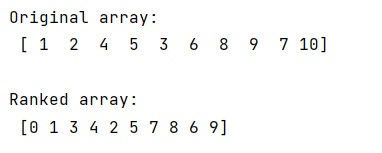# Rank items in an array using NumPy, without sorting array twice

Given a NumPy array, we have to rank items in an array using numpy without sorting array twice.
Submitted by Pranit Sharma, on December 28, 2022

NumPy is an abbreviated form of Numerical Python. It is used for different types of scientific operations in python. Numpy is a vast library in python which is used for almost every kind of scientific or mathematical operation. It is itself an array which is a collection of various methods and functions for processing the arrays.

## Ranking NumPy array items without sorting array twice

Suppose we are given an array and we need to create another array that represents the rank of each item in the first array.

For this purpose, we will use argsort() twice, first to obtain the order of the array, then to obtain ranking.

When dealing with 2D (or higher dimensional) arrays, we just need to be sure to pass an axis argument to argsort() to order over the correct axis.

Let us understand with the help of an example,

## Python code to rank items in an array using NumPy, without sorting array twice

```# Import numpy
import numpy as np

# Creating a numpy arrays
arr = np.array([1,2,4,5,3,6,8,9,7,10])

# Display original array
print("Original array:\n",arr,"\n")

# Ranking the array elements
ord = arr.argsort()
ranks = ord.argsort()

# Display result
print("Ranked array:\n",ranks)
```

Output: